# Control Systems - Online Test

Q1. Solution of the variables x1 and x2 for the following equations is to be obtained by employing the Newton-Raphson iterative method.
equation (i)    10x2 sinx1 - 0.8 = 0
equation (ii)    10x2 - 10x2 cosx1 - 0.6 = 0
Assuming the initial valued x1 = 0.0 and x2 = 1.0, the jacobian matrix is
Explaination / Solution: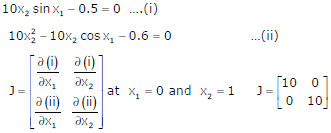Q2.
An open loop system represented by the transfer functionis
Explaination / Solution:

Open loop system stability is depends only on pole locations ⇒ system is stable There is one zero on right half of s-plane so system is non – minimum phase

Q3.
The frequency response of a linear system G(jw) is provided in the tubular form belowExplaination / Solution: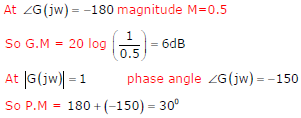Q4. A point Z has been plotted in the complex plane, as shown in figure below.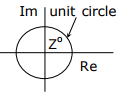The plot of the complex number y = 1/z is
Explaination / Solution: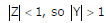Z is having +ve real part and positive imaginary part (from the characteristics) So Y should have +ve real part and negative imaginary part.

Q5.
The open loop transfer function G(s) of a unity feedback control system is given asFrom the root locus, it can be inferred that when k tends to positive infinity,
Explaination / Solution: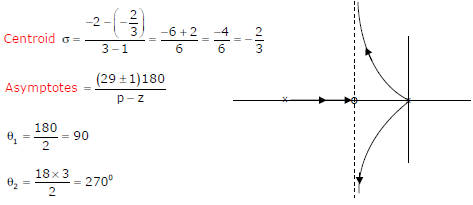Q6. A two loop position control system is shown below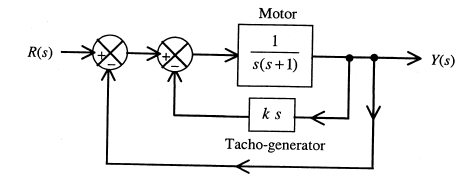The gain k of the Tacho-generator influences mainly the
Explaination / Solution: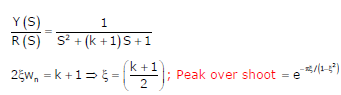Q7. If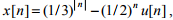then the region of convergence (ROC) of its Z-transform in the Z-plane will be
Explaination / Solution: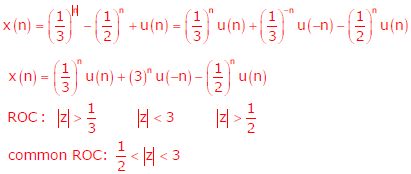Q8. Given. If C is a counterclockwise path in the z-plane such that |z + 1| = 1, the value ofis
Explaination / Solution:Q9. The unilateral Laplace transform of f (t) is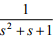. The unilateral Laplace transform of t f (t) is
Explaination / Solution:Q10. T he feedback system shown below oscillates at 2 rad/s when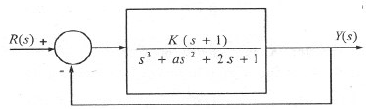Explaination / Solution: